##### Statistics For Dummies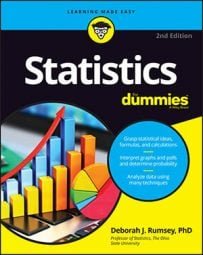You can test for an average difference using the paired t-test when the variable is numerical (for example, income, cholesterol level, or miles per gallon) and the individuals in the statistical sample are either paired up in some way according to relevant variables such as age or perhaps weight, or the same people are used twice (for example, using a pre-test and post-test).

Paired tests are typically used for studies in which someone is testing to see whether a new treatment, technique, or method works better than an existing method, without having to worry about other factors about the subjects that may influence the results.

With the average difference, you match up the subjects so they are thought of as coming from a single population, and the set of differences measured for each subject (for example, pre-test versus post-test) are thought of as one sample. The hypothesis test then boils down to a test for one population mean.

For example, suppose a researcher wants to see whether teaching students to read using a computer game gives better results than teaching with a tried-and-true phonics method. She randomly selects 20 students and puts them into 10 pairs according to their reading readiness level, age, IQ, and so on. She randomly selects one student from each pair to learn to read via the computer game method (abbreviated CM), and the other in the pair learns to read using the phonics method (abbreviated PM). At the end of the study, each student takes the same reading test. The data are shown in the following table.

Student Pair Computer Method Phonics Method Difference (CM – PM) Reading Scores for Computer Game Method versus Phonics Method 1 85 80 +5 2 80 80 0 3 95 88 +7 4 87 90 –3 5 78 72 +6 6 82 79 +3 7 57 50 +7 8 69 73 –4 9 73 78 –5 10 99 95 +4

The original data are in pairs, but you’re really interested only in the difference in reading scores (computer reading score minus phonics reading score) for each pair, not the reading scores themselves. So the paired differences (the differences in the pairs of scores) are your new data set. You can see their values in the last column of the table.

By examining the differences in the pairs of observations, you really only have a single data set, and you only have a hypothesis test for one population mean. In this specific example, the null hypothesis is that the mean (of the paired differences) is 0, and the alternative hypothesis is that the mean (of the paired differences) is greater than 0.

If the two reading methods are the same, the average of the paired differences should be near 0. If the computer method is better, the average of the paired differences should be significantly more than 0; that is, the computer reading score would be larger than the phonics score.

The notation for the null hypothesis is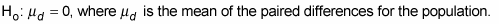(The d in the subscript just reminds you that you’re working with the paired differences.)

The formula for the test statistic for paired differences is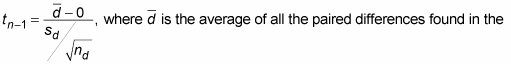sample, and tn–1 is a value on the t-distribution with nd – 1 degrees of freedom.

You use a t-distribution here because in most matched-pairs experiments the sample size is small and/or the population standard deviation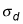is unknown, so it’s estimated by sd.

To calculate the test statistic for paired differences, do the following:

1. For each pair of data, take the first value in the pair minus the second value in the pair to find the paired difference.

Think of the differences as your new data set.

2. Calculate the mean,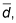and the standard deviation, sd, of all the differences.

3. Letting nd represent the number of paired differences that you have, calculate the standard error: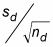4. Divide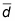by the standard error from Step 3.

Because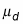is equal to 0 if H0 is true, it doesn’t really need to be included in the formula for the test statistic. As a result, you sometimes see the test statistic written like this: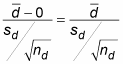For the reading scores example, you can use the preceding steps to see whether the computer method is better in terms of teaching students to read.

To find the statistic, follow these steps:

1. Calculate the differences for each pair (they’re shown in column 4 of the above table). Notice that the sign on each of the differences is important; it indicates which method performed better for that particular pair.

2. Calculate the mean and standard deviation of the differences from Step 1. In this example, the mean of the differences is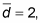and the standard deviation is sd = 4.64. Note that nd = 10 here.

3. The standard error is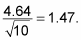(Remember that here, nd is the number of pairs, which is 10.)

4. Take the mean of the differences (Step 2) divided by the standard error of 1.47 (Step 3) to get 1.36, the test statistic.

Is the result of Step 4 enough to say that the difference in reading scores found in this experiment applies to the whole population in general? Because the population standard deviation,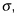is unknown and you estimated it with the sample standard deviation (s), you need to use the t-distribution rather than the Z-distribution to find your p-value. Using the below t-table, you look up 1.36 on the t-distribution with 10 – 1 = 9 degrees of freedom to calculate the p-value.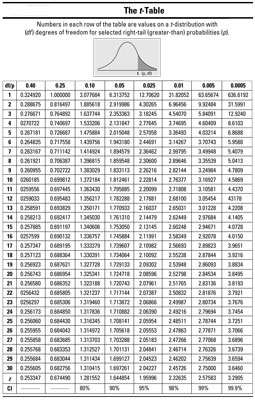The p-value in this case is greater than 0.10 (and hence greater than 0.05) because 1.36 is smaller than (or to the left of) the value of 1.38 on the table, and therefore its p-value is more than 0.10 (the p-value for the column heading corresponding to 1.38).

Because the p-value is greater than 0.05, you fail to reject H0; you don’t have enough evidence that the mean difference in the scores between the computer method and the phonics method is significantly greater than 0. However, that doesn’t necessarily mean a real difference isn’t present in the population of all students. But the researcher can’t say the computer game is a better reading method based on this sample of 10 students.

You might ask, “Hey, the sample mean of the differences is 2.0 which shows that the computer method was better than the phonics method . Why did the hypothesis test reject H0 since 2.0 is obviously greater than 0?” Because in this case, 2.0 is not significantly greater than 0. You also need to factor in variation using the standard error and the t distribution to be able to say something about the entire population of students.